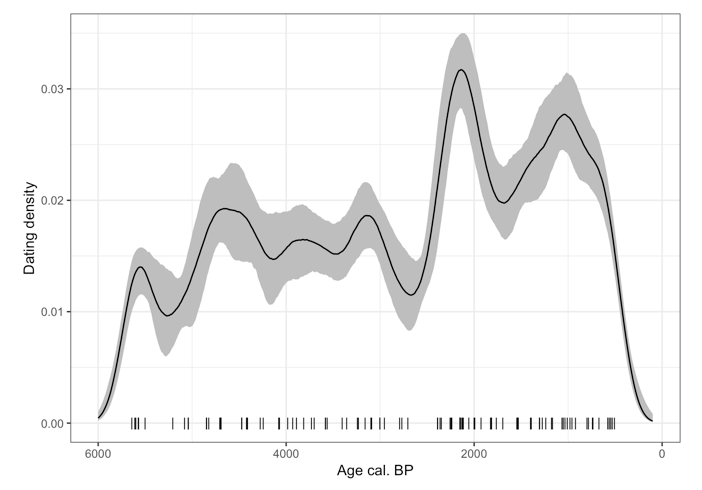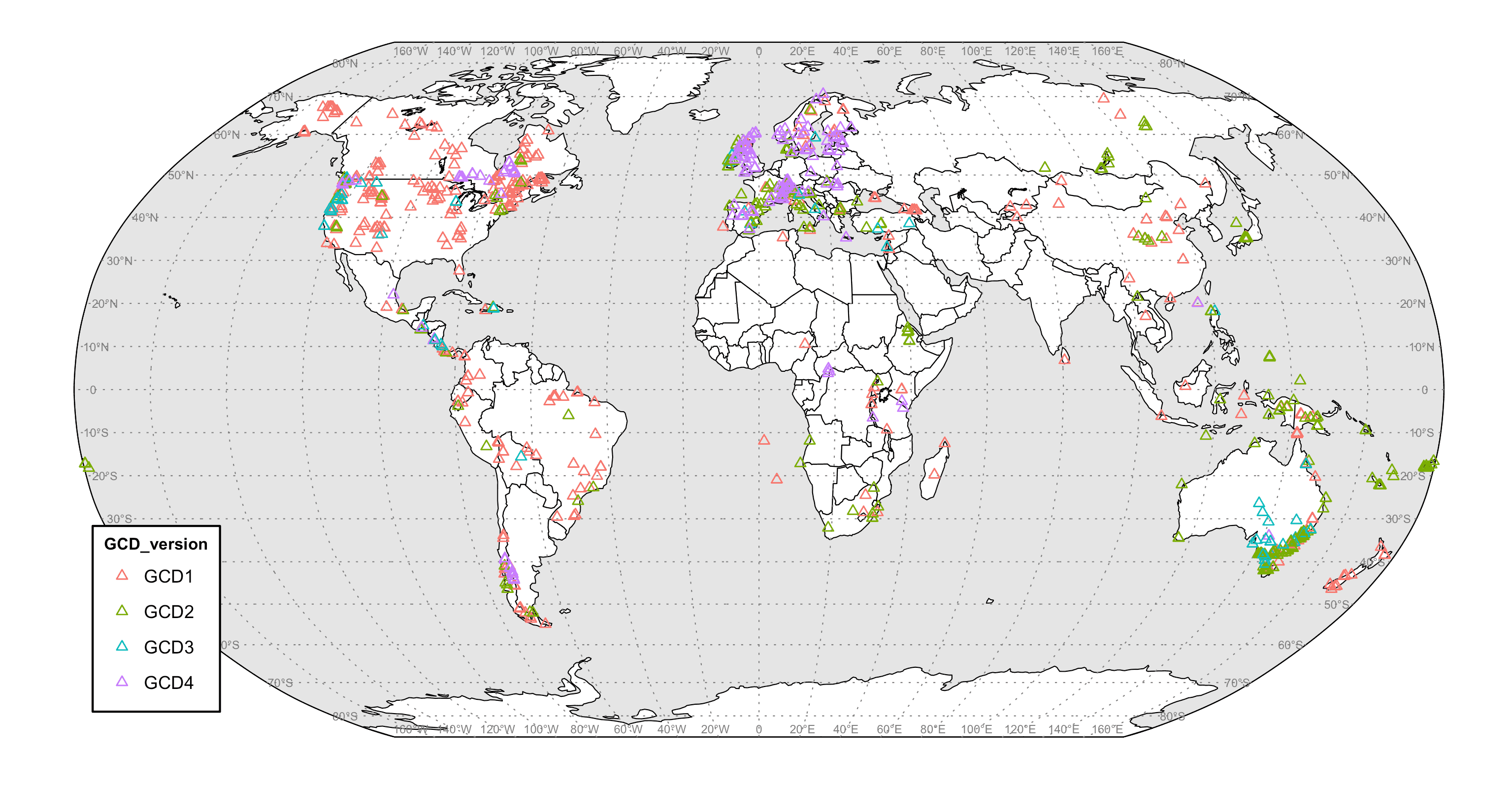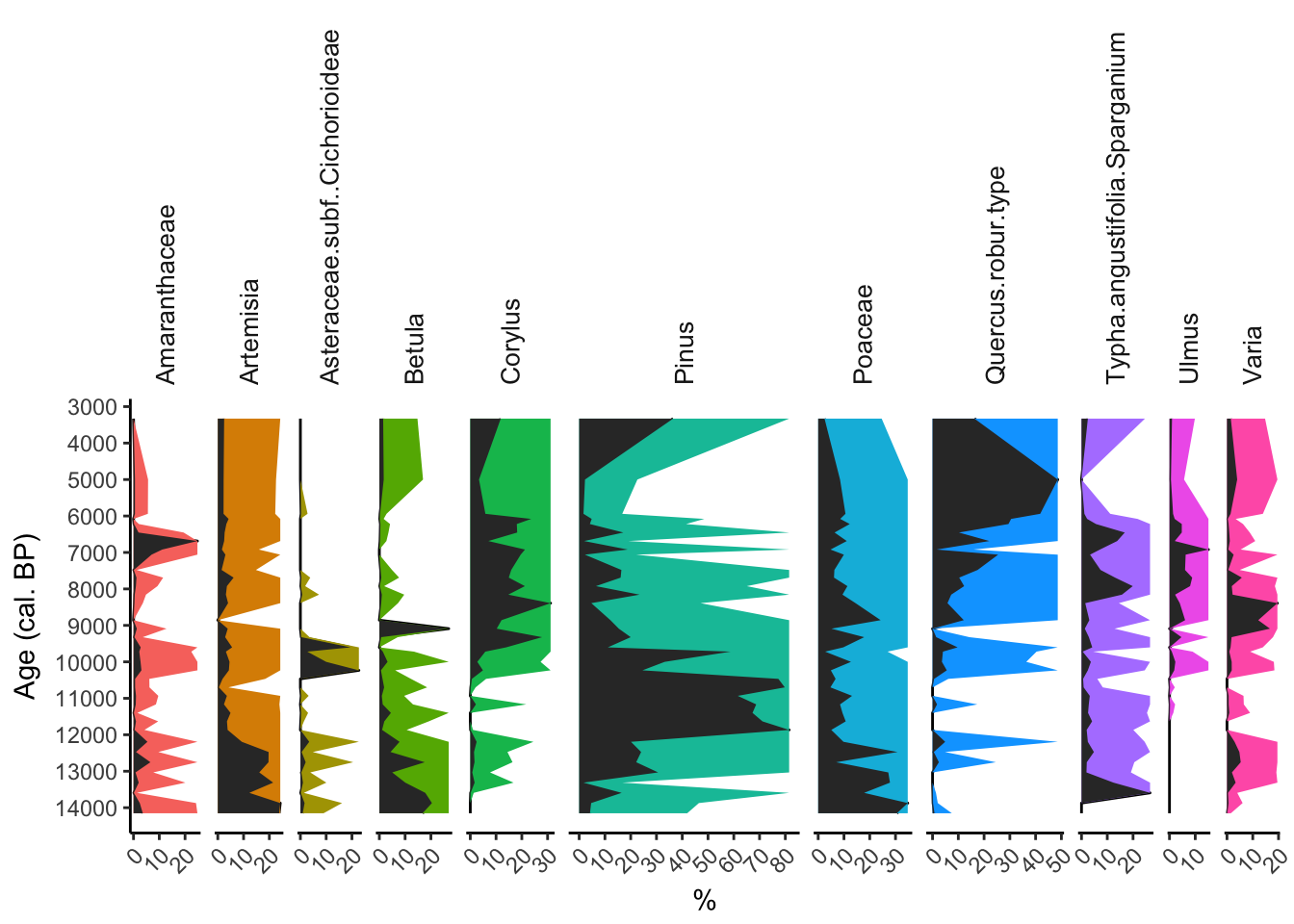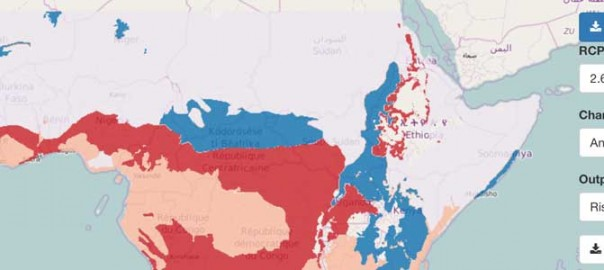## Temporal density of 14C dating

In this tutorial I illustrate the methods that have been used to reconstruct the curve of the temporal density of archeological dating around the Folly peat bog in Blarquez et al. (2018).

The approach is inspired by ‘clam’ package age-depth modeling (Blaauw, 2010). For each archeological dating, we will randomly pick one age according to its calibrated probability density (i.e., ages with a higher probability are more likely to be selected). We will then use a kernel density function to assess the temporal density of archeological dating. We will repeat this procedure 1000 times to assess the 95% confidence interval (CI) around the median trend in archeological sites dating during the Holocene.

In the original paper the archeological 14C dates came from the CARD database for sites that were located within a 200 km radius of Folly peat bog (Martindale et al., 2016), and wer completed with an additional set of 7 dates from an archeological site in Gatineau, which had yet to be included in the database (Archeotec, 2014). In this example we will simply simulate some 14C dating:

# rm(list=ls())
library(Bchron)
library(ggplot2)

## Generate a random list of 14C dating --------------------------------------------------
set.seed(12)
age_14C=round(runif(100, 40, 500))*10
age_sd=round(runif(100, 2, 7))*10


We then calibrate each date in the database using the IntCal13 calibration curve (Reimer et al., 2013):

## Calibrate these dates using Bchron ---------------------------------------------------

age=BchronCalibrate(ages=age_14C,
ageSds=age_sd,
calCurves=rep('intcal13',length(age_14C)))


Here we define a custom function that will be used to sample an age within each dates distribution. This sampling is done according to the probability density distribution of each age. This procedure is somewhat equivalent with clam and bacon sampling procedure.

## Sample one age with probability (equivalent to clam and ?bacon) -----------------------
## Use lapply to sample inside a list of ages

foo=function(x){
y=sample(x$ageGrid,1,prob=x$densities)
return(y)
}
# Test a single iteration
ragelist=lapply(age,foo)
dates=as.vector(unlist(ragelist));dates


Now we will run this sampling 1000 times and for each iteration we will reconstruct the density of calibrated ages using kernel density estimation with a bandwidth of 150 years. We will subsequently calculate the median density of ages and 95% confidence intervals.

n=length(age_14C) #number of events
age_=seq(100, 6000, 10); # adapt to your time frame

## Bootstrap --------------------------------------------------
nbboot=1000
fmat=matrix(nrow=length(age_),ncol=nbboot)

for(i in 1:nbboot){
ragelist=lapply(age,foo)
dates=as.vector(unlist(ragelist))
fmat[,i]=c(density(dates,
bw=150,kernel="gaussian",from=100,
to=6000, n=length(age_))$y*n) cat(i," ") } alpha=0.05 CI=apply(fmat, 1, quantile, probs=c(alpha/2,0.5,1-(alpha/2)) )  Plot the temporal trends in the density of archeological dating (along with max intercepts for each date sensu Telford et al. (2004), for display purpose only): ## Plot -------------------------------------------------------- bar=function(x){ z=x$ageGrid[which.max(x$densities)] return(z) } magelist=lapply(age,bar) mdates=as.vector(unlist(magelist)); df2=data.frame(x=mdates,y =0) df=data.frame(age=age_, t(CI)) ggplot()+ geom_ribbon(data=df,aes(x=age,ymax=X97.5.,ymin=X2.5.),fill="grey")+ geom_line(data=df,aes(x=age_,y=X50.))+ geom_point(data=df2,aes(x,y),pch="|",size=3)+ scale_x_reverse()+ ylab("Dating density")+xlab("Age cal. BP")+ theme_bw() ## --------------------------------------------------------------  Archéotec, 2014. Travaux de réaménagement de la rue Jacques-Cartier, ville de Gatineau Site BiFw-172, Blaauw, M., 2010. Methods and code for “classical” age-modelling of radiocarbon sequences. Quaternary Geochronology, 5(5), pp.512–518. Available at: http://dx.doi.org/10.1016/j.quageo.2010.01.002. Blarquez, O. et al., 2018. Late Holocene influence of societies on the fire regime in southern Québec temperate forests. Quaternary Science Reviews, 180, pp.63–74. Available at: http://linkinghub.elsevier.com/retrieve/pii/S0277379117307060 [Accessed December 15, 2017]. Martindale, A. et al., 2016. Canadian Archaeological Radiocarbon Database (CARD 2.1), accessed 15 September 2017. , 2004. Reimer, P.J. et al., 2013. IntCal13 and Marine13 Radiocarbon Age Calibration Curves 0–50,000 Years cal BP. Radiocarbon, 55(04), pp.1869–1887. Available at: https://www.cambridge.org/core/product/identifier/S0033822200048864/type/journal_article. Telford, R.J., Heegaard, E. & Birks, H.J.B., 2004. The intercept is a poor estimate of a calibrated radiocarbon age. The Holocene, 14(2), pp.296–298. Available at: http://journals.sagepub.com/doi/10.1191/0959683604hl707fa.## GCD 4.0.2 and paleofire 1.2.2 We are please to announce that the GCD and paleofire R packages have been updated to their 4.0.2 and 1.2.2 versions, respectively. GCD major update from 3.X.X to 4.X.X version number is associated to extensive changes in the way data is added into the package. GCD 4.X.X is now mirroring the online GCD SQL database on a monthly basis or whenever a significant number of new charcoal sites are added to the database. Three new fields in the paleofiresites table (see ?paleofiresites for details) enable to perform analyses with specific database versions or enable to select sites according to the date at which they have been added into the database. See the quick example below for explanations: par(mfrow=c(2,2)) plot( pfSiteSel(num_version < 400 ) ) # All sites in GCD version before 4.0.0 plot( pfSiteSel(gcd_version == "GCD1" ) ) # All sites in GCDv1 plot( pfSiteSel(update_date < "2016-01-01" ) ) # Sites included before 2016-01-01 plot( pfSiteSel(update_date > "2018-01-01" ) ) # Sites included since 2018-01-01The map above that is used to display all GCD charcoal sites has been produced by adapting the code available at https://seethedatablog.wordpress.com/2016/12/23/r-simple-world-map-robinson-ggplot/ please follow that link for additional references and explanations. The modified code is copied below: # ====================================================================================== # Create a simple world map in Robinson projection with labeled graticules using ggplot # ====================================================================================== # Set a working directory with setwd() or work with an RStudio project # __________ Set libraries library(rgdal) # for spTransform() &amp;amp; project() library(ggplot2) # for ggplot() library(paleofire) # setwd("somewhere...") # __________ Load ready to use data from GitHub load(url("https://github.com/valentinitnelav/RandomScripts/blob/master/NaturalEarth.RData?raw=true")) # This will load 6 objects: # xbl.X &amp;amp; lbl.Y are two data.frames that contain labels for graticule lines # They can be created with the code at this link: # https://gist.github.com/valentinitnelav/8992f09b4c7e206d39d00e813d2bddb1 # NE_box is a SpatialPolygonsDataFrame object and represents a bounding box for Earth # NE_countries is a SpatialPolygonsDataFrame object representing countries # NE_graticules is a SpatialLinesDataFrame object that represents 10 dg latitude lines and 20 dg longitude lines # (for creating graticules check also the graticule package or gridlines fun. from sp package) # (or check this gist: https://gist.github.com/valentinitnelav/a7871128d58097e9d227f7a04e00134f) # NE_places - SpatialPointsDataFrame with city and town points # NOTE: data downloaded from http://www.naturalearthdata.com/ # here is a sample script how to download, unzip and read such shapefiles: # https://gist.github.com/valentinitnelav/a415f3fbfd90f72ea06b5411fb16df16 # __________ Project from long-lat (unprojected data) to Robinson projection # spTransform() is used for shapefiles and project() in the case of data frames # for more PROJ.4 strings check the followings # http://proj4.org/projections/index.html # https://epsg.io/ PROJ &amp;lt;- "+proj=robin +lon_0=0 +x_0=0 +y_0=0 +ellps=WGS84 +datum=WGS84 +units=m +no_defs" # or use the short form "+proj=robin" NE_countries_rob &amp;lt;- spTransform(NE_countries, CRSobj = PROJ) NE_graticules_rob &amp;lt;- spTransform(NE_graticules, CRSobj = PROJ) NE_box_rob &amp;lt;- spTransform(NE_box, CRSobj = PROJ) # Add lakes http://www.naturalearthdata.com/http//www.naturalearthdata.com/download/10m/physical/ne_10m_lakes.zip download.file("http://www.naturalearthdata.com/http//www.naturalearthdata.com/download/10m/physical/ne_10m_lakes.zip", destfile="ne_10m_lakes.zip") unzip("ne_10m_lakes.zip") NE_lakes &amp;lt;- readOGR('ne_10m_lakes.shp', 'ne_10m_lakes') NE_lakes &amp;lt;- spTransform(NE_lakes, CRSobj = PROJ) NE_lakes &amp;lt;- NE_lakes[NE_lakes@data$scalerank==0,]

# project long-lat coordinates for graticule label data frames
# (two extra columns with projected XY are created)
prj.coord &amp;lt;- project(cbind(lbl.Y$lon, lbl.Y$lat), proj=PROJ)
lbl.Y.prj &amp;lt;- cbind(prj.coord, lbl.Y)
names(lbl.Y.prj)[1:2] &amp;lt;- c("X.prj","Y.prj")

prj.coord &amp;lt;- project(cbind(lbl.X$lon, lbl.X$lat), proj=PROJ)
lbl.X.prj &amp;lt;- cbind(prj.coord, lbl.X)
names(lbl.X.prj)[1:2] &amp;lt;- c("X.prj","Y.prj")

# Project GCD sites

gcd_rob=project(cbind(paleofiresites$long,paleofiresites$lat),PROJ)
gcd=data.frame(long=gcd_rob[,1],lat=gcd_rob[,2],GCD_version=paleofiresites$gcd_version) # __________ Plot layers ggplot() + # add Natural Earth countries projected to Robinson, give black border and fill with gray geom_polygon(data=NE_box_rob, aes(x=long, y=lat), colour="black", fill="grey90", size = 0.25) + geom_polygon(data=NE_countries_rob, aes(long,lat, group=group), colour="black", fill="white", size = 0.25) + geom_polygon(data=NE_lakes, aes(long,lat, group=group), colour="black", fill="white", size = 0.25) + # Note: "Regions defined for each Polygons" warning has to do with fortify transformation. Might get deprecated in future! # alternatively, use use map_data(NE_countries) to transform to data frame and then use project() to change to desired projection. # add Natural Earth box projected to Robinson geom_polygon(data=NE_box_rob, aes(x=long, y=lat), colour="black", fill="transparent", size = 0.25) + # add graticules projected to Robinson geom_path(data=NE_graticules_rob, aes(long, lat, group=group), linetype="dotted", color="grey50", size = 0.25) + # add graticule labels - latitude and longitude geom_text(data = lbl.Y.prj, aes(x = X.prj, y = Y.prj, label = lbl), color="grey50", size=2) + geom_text(data = lbl.X.prj, aes(x = X.prj, y = Y.prj, label = lbl), color="grey50", size=2) + # the default, ratio = 1 in coord_fixed ensures that one unit on the x-axis is the same length as one unit on the y-axis geom_point(data=gcd, aes(long,lat, col= GCD_version),pch=2)+ coord_fixed(ratio = 1) + # remove the background and default gridlines theme_void()+ theme(legend.title = element_text(colour="black", size=8, face="bold"), # adjust legend title legend.position = c(0.1, 0.2), # relative position of legend plot.margin = unit(c(t=0, r=0, b=0, l=0), unit="cm"), legend.background = element_rect(fill="white", size=0.5, linetype="solid", colour ="black")) # adjust margins # save to pdf and png file ggsave("map_draft_1.pdf", width=28, height=13.5, units="cm") ggsave("map_1.png", width=26, height=13.5, units="cm", dpi=300) # REFERENCES: # This link was useful for graticule idea # http://stackoverflow.com/questions/38532070/how-to-add-lines-of-longitude-and-latitude-on-a-map-using-ggplot2 # Working with shapefiles, projections and world maps in ggplot # http://rpsychologist.com/working-with-shapefiles-projections-and-world-maps-in-ggplot## ggplot2 pollen diagram (and dendrogram) In R, excellent packages provide ways to plot pollen or more generally stratigraphic diagrams. The two main tools come from the rioja package with “strat.plot” and the analogue package with “Stratiplot”. Althought those two functions are very comprehensive (you can include a dendrogram, pollen zones, etc.), easy to use, and highly customizable; I was still wondering if there is a way in R to plot a simple pollen diagram using only general plot syntax an preferably ggplot2. I came up with this simple solution that involve only ggplot2 syntax. Probably there is a possibility to add a dendrogram with grid.arrange and to overlay additionnal information but right now this approach seems sufficient to cover my very basic plotting needs: library(ggplot2) library(grid) library(neotoma) library(analogue) ## Loading required package: vegan ## Loading required package: permute ## Loading required package: lattice ## This is vegan 2.4-1 ## analogue version 0.17-0  First extract data from Neotoma using neotoma package, we will have a look at “Le Grand Etang de Suze-La-Rousse” in Provençal Drome by: “Argant, J. 1990. Climat et environnement au Quaternaire dans le bassin du Rhône d’après les données palynologiques. Documents du Laboratoire de Géologie de Lyon 3:1-199.” suze <- get_site(sitename = 'Le Grand Etang%') suze_pollen=get_dataset(suze) suze_data=get_download(suze_pollen) # head(suze_data[]$counts)

core.pct <- data.frame(tran(suze_data[]$counts, method = 'percent')) age <- suze_data[]$sample.meta$age core.pct <- chooseTaxa(core.pct, max.abun = 10) Stratiplot(age ~ ., core.pct, sort = 'wa', type = 'poly', ylab ='Years Before Present')Now we create a simple data.frame with information for plotting a pollen diagram in ggplot2: df=data.frame(yr=rep(age,ncol(core.pct)), per=as.vector(as.matrix(core.pct)), taxa=as.factor(rep(colnames(core.pct),each=nrow(core.pct))))  We define a theme without gridlines, etc. and elements that will decrease readability of the diagram theme_new <- theme(panel.grid.major = element_blank(), panel.grid.minor = element_blank(), # remove grids panel.background = element_blank(), axis.line = element_line(colour = "black"), strip.text.x = element_text(size=10, angle=90, vjust=0), # Taxa names strip.background = element_blank(), strip.text.y = element_text(angle = 0), legend.position="none",panel.border = element_blank(), axis.text.x=element_text(angle=45,hjust=1)) # Axis tick label angle  And finally the pollen diagram: ggplot(df)+ geom_line(aes(yr,per))+ geom_area(aes(yr,per))+ scale_x_reverse(breaks =seq(0,100000,1000))+ scale_y_continuous(breaks =seq(0,100,10))+ xlab("Age (cal. BP)")+ylab("%")+ coord_flip()+ theme_new+ facet_grid(~df$taxa,scales = "free", space = "free")Add an exageration line (the quick and dirty way):

df$exag=df$per*10
id=unique(df$taxa) for(i in 1:length(id)){ uplim=which(df$exag[df$taxa==id[i]]>max(df$per[df$taxa==id[i]])) df$exag[df$taxa==id[i]][uplim]<-max(df$per[df$taxa==id[i]]) } diag=ggplot(df)+ geom_line(aes(yr,per))+ geom_area(aes(yr,exag,fill=taxa))+ geom_area(aes(yr,per))+ scale_x_reverse(breaks =seq(0,1e8,1000))+ scale_y_continuous(breaks =seq(0,100,10))+ xlab("Age (cal. BP)")+ylab("%")+ coord_flip(xlim=c(3000,14000))+ theme_new+ facet_grid(~df$taxa,scales = "free", space = "free")

diagBecause only ggplot2 syntax is used you can play around and add geom_point, geom_bar layouts etc. and customize your diagram the way you want with the theme() function.

UPDATE: Adding a dendrogram is not straightforward but definitively doable. We will use a combinason of rioja and ggdendro package such as:

diss <- dist(sqrt(core.pct/100)^2)
clust <- chclust(diss, method="coniss")
# bstick(clust) # optionnally look at number of optimal zones


Now we will use ggdendro and modify its output in order to inject ages that will replace sample numbers:

dendro <- as.dendrogram(clust)
ddata <- dendro_data(dendro, type="rectangle")

## MOD the segment data frame for age instead of value order -----------------------
ddata$segments->yo yo$xx=NA
yo$xxend=NA for(i in 1:length(age)){ yo$xx[which(yo$x == i)]=age[i] yo$xxend[which(yo$xend == i)]=age[i] da=age[i+1]-age[i] yo$xx[yo$x>i & yo$x<i+1]<-age[i]+(yo$x[yo$x>i & yo$x<i+1]-i)*da yo$xxend[yo$xend>i & yo$xend<i+1]<-age[i]+(yo$xend[yo$xend>i & yo$xend<i+1]-i)*da } # Please dont ask.... ddata$segments$x<-yo$xx
ddata$segments$xend<-yo$xxend # -----------------------------------------------------------------------------------  We finally can plot the dendrogram along with the diagram with gridExtra dendro=ggplot(segment(ddata)) + geom_segment(aes(x=x, y=y, xend=xend, yend=yend)) + coord_flip(xlim=c(3000,14000)) + ylab("Distance")+ scale_x_reverse(breaks =seq(0,1e8,1000))+ theme_new+theme(axis.title.y=element_blank(), axis.text.y=element_blank(), axis.ticks.y=element_blank(), axis.line.y = element_blank()) g1 = ggplotGrob(diag) g2 = ggplotGrob(dendro) g2$heights[5:7]<-g1heights[6:8] # Important to align plots! grid.arrange(g1, g2, widths =c(1,0.3),ncol=2,newpage = TRUE)You can follow the overall logic to add any type of additional plot as long as its a ggplot2 object! ## paleofire 1.1.9 ! A new version of the R package paleofire 1.1.9 is available on CRAN (https://CRAN.R-project.org/package=paleofire) and GitHub (https://github.com/oblarquez/paleofire). The release includes few bug fixes and improvements of existing functions, including more flexibility in pfAddData function and new options in kdffreq, see examples below: library(paleofire) # Control the type and format of user defined charcoal files # here for examples csv files with three columns #(depth, age and char) separated with semicolon and with "." # character used in the file for decimal points: head(read.csv("http://blarquez.com/public/data/Ben_area.csv" ,sep=";")) files=c("http://blarquez.com/public/data/Ben_area.csv", "http://blarquez.com/public/data/Small_area.csv") mydata=pfAddData(files=files, sep=";", dec=".") # Transform and compositing: TR=pfTransform(add=mydata, method=c("MinMax","Box-Cox","Z-Score"), BasePeriod=c(200,2000)) COMP=pfComposite(TR1, bins=seq(0,8000,500)) plot(COMP)# Estimate the frequency of armed conflicts from 1946 to 2014 # using kernel density estimation from kdffreq # Data from the The Uppsala Conflict Data Program (UCDP) available at: https://www.prio.org dat=read.csv('http://ucdp.uu.se/downloads/ucdpprio/ucdp-prio-acd-4-2016.csv') res=kdffreq(datYear,bandwidth = "bw.ucv", nbboot=1000, up = 1946, lo = 2014, interval=1, pseudo=T)
plot(res, ylab="# armed conflict/year")## Data: Land-use change outweighs projected effects of changing rainfall on tree cover in sub-Saharan Africa

Click to access to data!

Data citation: Aleman J. C., Blarquez O., Staver A. C. 2016. Land-use change outweighs projected effects of changing rainfall on tree cover in sub-Saharan Africa. Global Change Biology DOI: 10.1111/gcb.13299

## Data: Tree biomass reconstruction shows no lag in post-glacial afforestation of eastern Canada

Data from: Blarquez O. and J. Aleman. Tree biomass reconstruction shows no lag in post-glacial afforestation of eastern Canada. Canadian Journal of Forest Research. DOI: 10.1139/cjfr-2015-0201

You can use the application below for viewing and downloading specific time slice data. The shiny application is also available here, all data are zipped here. Simply decompress the archive, then in R use for example:

load('acer_50km_data_8000BP.rda')
# plot the raster object
plot(gr$raster) # view data head(gr$df)
# check projection
gr$proj4 # gr is an object of the class "pfGridding" # from the paleofire package and can be manipulated # and modified using the plot function: library(paleofire) ?plot.pfGridding p=plot(gr,continuous=F,col_class=c(0,5,10,15), cpal = "Purples", anomalies=FALSE, points=TRUE, empty_space = 5) p  ## paleofire R presentation paleofire R package presentation given at the Global Paleofire Working Group workshop at Harvard Forest the 28 september 2015. Paleofire: data-model comparisons for the past millenium. paleofire_R.pdf ## paleofire 1.1.5 A new version of the R package paleofire is available on CRAN at http://cran.r-project.org/web/packages/paleofire/index.html This release includes a new vignette on the basic usage of paleofire for reconstructing regional charcoal composite curves and a new function to easily export GCD sites to Google Earth .kml file.The australasian sites above have been exported using: library(paleofire) x=pfSiteSel(id_region=="AUST") require(sp) pfToKml(x, file='/Users/Olivier/Desktop/sites.kml')  ## paleofire package highlighted in the PAGES Magazine The paleofire package has been highlighted in the April issue of the PAGES Magazine :Vannière B., O. Blarquez, J. Marlon, A.-L. Daniau and M. Power. 2014. Multi-Scale Analyses of fire-climate- Vegetation Interactions on Millennial Scales. PAGES Magazine, Volume 22, Page 40. The article presents the workshop of the Global Paleofire Working Group held in Frasne (France) on 2-6 October 2013 that was supported by PAGES. The pdf of the article can be downloaded here or directly from the PAGES website. ## Introducing the paleofire package The new R package, paleofire, has been released on CRAN. The package is dedicated to the analysis and synthesis of charcoal series contained in the Global Charcoal Database (GCD) to reconstruct past biomass burning. paleofire is an initiative of the Global Paleofire Working Group core team, whose aim is to encourage the use of sedimentary charcoal series to develop regional to global synthesis of paleofire activity, and to ease access to the GCD data through a common research framework that will be useful to the paleofire research community. Currently, paleofire features are organized into three different parts related to (i) site selection and charcoal series extraction from GCD data; (ii) charcoal data transformation and homogenization; and (iii) charcoal series compositing and syntheses. Installation: paleofire is available from both CRAN website and GitHub To install the official release (v1.0, 01/2014) from CRAN you just need to type this line at the R prompt:  install.packages("paleofire") To install the development version of paleofire from the GitHub repository the devtools package is required: on Windows platform the Rtools.exe program is required in order to install the devtools package. Rtools.exe can be downloaded for a specific R version on http://cran.r-project.org/bin/windows/Rtools/ Once devtools is installed type the following lines at R prompt to install paleofire: library(devtools) install_github("paleofire","paleofire") library(paleofire)  To test everything is working you can plot a map of all charcoal records included in the Global Charcoal Database v03: plot(pfSiteSel())  For details and examples about paleofire please refer to the included manual. More examples of the capabilities of paleofire to come in the near future… ## Calculate pixel values at increasing radius ndvi_classes.m function could be used to calculate the number and percentage of pixels at increasing radius from a lake following certain criterion’s. The function was originally developed to count the number of pixels falling in specific NDVI classes around lakes but may be useful for different proxies and situations. The function use georeferenced tiff images as input. For details please refer to Aleman et al. (2013) or to the included help. The image used in the example can be downloaded here.The function: function [classe,classe_per]=ndvi_classes(ImageName,critere1,critere2) % INPUT: ImageName is the name of the geotiff image for imput % critere1 (and 2) criterion for classes evaluation % % OUTPUT: classe: vector of NDVI classes at increasing radius from % the lake center (number of pixel falling in class) % classe_per: Same as classe but in percentage of pixels % EXAMPLE: Using the given Image 'classif_GBL_94.tif' %{ [classe classe_per]=ndvi_classes('classif_GBL_94.tif','>2','<4'); %Plot the classe as a function of distance in km %(1pixel=0.0301km, input 200 pixels when asked) plot((1:200)*0.0301,classe_per,'k-') ylabel('% pixels >2 and <4') xlabel('Distance from lake center (km)') %} % Blarquez Olivier 2012 % blarquez@gmail.com [Imge,dat] = geotiffread(ImageName); imagesc(double(Imge)) select= input('Select point interactively? Y/N: ', 's'); if select=='Y' title('Please double clic on lake center:') p = impoint(gca,[]); p = wait(p); p=round(p); elseif select=='N' valstring = input('Enter latitude and longitude: ', 's'); valparts = regexp(valstring, '[ ,]', 'split'); coords = str2double(valparts); [~,p(2)]=min(abs(linspace(dat.Latlim(1),dat.Latlim(2),dat.RasterSize(1))-... coords(1))); [~,p(1)]=min(abs(linspace(dat.Lonlim(1),dat.Lonlim(2),dat.RasterSize(2))-... coords(2))); end radius = input('Radius in pixels to be evaluated: '); Imge(:,((p(1)+(radius)):end))=[]; Imge(:, 1:(p(1)-(radius)))=[]; Imge(((p(2)+(radius)):end),:)=[]; Imge(1:(p(2)-(radius)),:)=[]; n=length(Imge); classe=zeros(radius,1); classe_per=zeros(radius,1); for r=1:radius warning('off','all') imshow(Imge) %imagesc(double(Imge)) hEllipse = imellipse(gca,[(n/2-(r)) n/2-(r) r*2 r*2]); maskImage = hEllipse.createMask(); imshow(maskImage); maskedImge = Imge .* cast(maskImage,class(Imge)); %imshow(maskedImge); maskedImge(maskedImge==0)=NaN; pixList=reshape(maskedImge,[],1); pixList(isnan(pixList),:)=[]; classe(r,1)=length(pixList(eval(['pixList',critere1]) & ... eval(['pixList',critere2]),:)); classe_per(r,1)=classe(r,1)./length(pixList(:,1)); end close all warning('on','all') end  ## Paleofire reconstruction based on an ensemble-member strategy Matlab codes and data associated with the manuscript: Blarquez O., M. P. Girardin, B. Leys, A. A. Ali, J. C. Aleman, Y. Bergeron and C. Carcaillet. 2013. Paleofire reconstruction based on an ensemble-member strategy applied to sedimentary charcoal. Geophysical Research Letters 40: 2667–2672, are available here. Preprocessed data for Lac à la Pessière and Lago perso used in the paper also available: PESSIERE.mat.zip PERSO.mat.zip ## Replicated rarefied richness This example is intended to present the methods develloped for the paper Blarquez et al. (2013). We will use the macroremain record of the Lac du Loup a small subalpine lake with continuous macoremain counts for the last 11 750 years. More details on this site can be found in Blarquez et al. (2010). First load the macroremain data: dataMacro=read.csv("http://blarquez.com/public/code/dataMacro.csv") # Macroremain counts paramMacro=read.csv("http://blarquez.com/public/code/paramMacro.csv") # Associated depths, ages and sample volumes dataMacro[is.na(dataMacro)]=0 # Set missing values to zero  We now caluculates the median resolution of the record and the interpolated ages at which the influx will be calculated: resMed=median(diff(paramMacro[,3])) Ages=seq(-50, 11750, resMed)  We reconstruct the influx matrix using the pretreatment function which is the R implementation of the CharAnalysis CharPretreatment.m function originally develloped by P. Higuera and available at https://sites.google.com/site/charanalysis Requires a matrix as input with the following columns: CmTop CmBot AgeTop AgeBot Volume (params) A serie from which to calculate accumulation rates (serie) source("http://blarquez.com/public/code/pretreatment.R") infMacro=matrix(ncol=length(dataMacro[1,]),nrow=length(Ages)) for (i in 1:length(dataMacro[1,])){ + infMacro[,i]=c(pretreatment(params=paramMacro,serie=dataMacro[,i], + yrInterp=resMed,first=-50,last=11750, + plotit=F,Int=F)$accI)
+ }



Calculate samples macroremain sums and the minimun influx sum:

S=rowSums(infMacro)
nMin=min(na.omit(S[1:length(S)-1]))



We exclude the last sample (not calculated because of unknown accumulation rate) and samples with NA (because of vol==0):

del=which(S==0 | is.na(S))
infMacro=infMacro[-del,]
Ages=Ages[-del]
S=S[-del]



We calculate a proportion matrix which is used to replicate the rarefaction procedure:

S_=matrix(ncol=ncol(infMacro),nrow=nrow(infMacro))
for (i in 1:length(infMacro[1,])){
+ S_[,i]=c(S)
+ }
propMat=infMacro/S_



Replicate rarefaction with min influx n=1,2,…,500.
We will use the rarefy function from the package vegan:

library(vegan)
rare_=matrix(nrow=nrow(infMacro),ncol=500)
for (n in 1:500){
+ infN=ceiling(S_*n/nMin*propMat)
+ rare_[,n]=c(rarefy(infN,sample=n))
+ }



And finnally calculates the 90 percent confidence intervals and plot the rarefied richness using a 500 years locally weighted scatter plot smoother:

CI_=t(apply(rare_, 1, quantile, probs = c(0.05, 0.5, .95), na.rm = TRUE))
span=500/resMed/length(Ages)
plot(Ages,lowess(CI_[,2], f =span)$y,type="l",ylab="E[Tn]",ylim=c(min(CI_), + max(CI_)),xlab="Age",main="Rarefied Richness",xlim=c(12000,-100)) lines(Ages,lowess(CI_[,1], f =span)$y,type="l",lty=2)
lines(Ages,lowess(CI_[,3], f =span)\$y,type="l",lty=2)References:
Blarquez O., Finsinger W. and C. Carcaillet. 2013. Assessing paleo-biodiversity using low proxy influx. PLoS ONE 8(4): e65852
Blarquez O., Carcaillet C., Mourier B., Bremond L. and O. Radakovitch. 2010. Trees in the subalpine belt since 11 700 cal. BP : origin, expansion and alteration of the modern forest. The Holocene 20 : 139-146. DOI : 10.1177/0959683609348857

Download pdf vignette

## Superposed Epoch Analysis (SEA)

SEA.m function for Superposed Epoch Analysis (SEA) with confidence intervals estimated using block bootstrap procedure, the method is freely inspired from Adams et al. (2003), for an example of using SEA in palaeoecology see Blarquez and Carcaillet (2010). For details please refer to included help.

Example:

Create a random time serie with noise, define some events and plot them along with the time series:

t=1:500;
d=2.5*sin(2*pi*t/100)+1.5*sin(2*pi*t/41)+1*sin(2*pi*t/21);
T=(d+2*randn(size(d))).';
figure()
plot(t,T,'g')
ev=[50,93,131,175,214,257,297,337,381,428,470].';
hold on
plot(ev,repmat(4,length(ev),1),'v')Then we perform a SEA using a time window of 20 years before and 20 years after each event. ‘nbboot’ argument is used to define the number of circular block bootsrtap replicates with an automatically calculated block length (‘b’ equal 0) following Adams et al. (2003) procedure.


[SEA_means,SEA_prctiles]=SEA(T,ev,20,20,'prctilesIN',[5,95],'nbboot',9999,'b',0)Proxy response to the randomly generated events appears significant at the 95% confidence levels during events occurrence (at a lag close to zero).

## Paleofire frequencies

KDffreq.m function calculates palaeo-fire frequency using a Gaussian kernel and computes bootstrapped confidence intervals arround fire frequency. Require the date of fire events as input variable. Associated with this function analyst may use cvKD.mfunction to estimate optimal kernel bandwidth by max log likelihood. Here’s an example:

Generate some fire dates for the last 8000 years:

x=randi(8000,40,1);
plot(x,1,'+','col','red')
set(gca,'xdir','reverse')
xlabel('Time (cal yrs. BP)')Use the cvKD.m function to calculate the optimal bandwidth for the kernel:

cvKD(x)
ans = 724.3434


Then we estimate the long term fire frequency using the Gaussian kernel density procedure:

 [ffreq]=KDffreq(x,'up',0,'lo',8000,'bandwidth',700,'nbboot',100,'alpha',0.05 );